# Changes to the Mathematical Language of Event-B

This document describes the evolution of the Event-B mathematical language that happened in release 1.0.0. See Event-B_Mathematical_Language for a full description of the language.

## Generic Identity and Projections

Three operators were still unary while they could be atomic and generic:

• the identity relation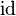$\id$
• the first projection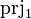$\prjone$
• the second projection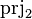$\prjtwo$

These operators are defined as follows in the old version: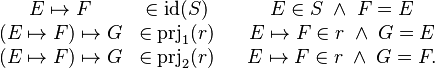$\begin{matrix} E\mapsto F &\in\id(S) && E\in S\;\land\; F = E\\ (E\mapsto F)\mapsto G &\in\prjone(r) && E\mapsto F\in r\;\land\; G = E\\ (E\mapsto F)\mapsto G &\in\prjtwo(r) && E\mapsto F\in r\;\land\; G = F . \end{matrix}$


If we drop the parameter, we get much more straightforward definitions that capture the essence of the operator. The new definitions are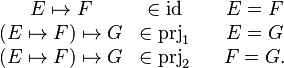$\begin{matrix} E\mapsto F &\in\id && E = F\\ (E\mapsto F)\mapsto G &\in\prjone && E = G\\ (E\mapsto F)\mapsto G &\in\prjtwo && F = G . \end{matrix}$


We have the following equivalence between the old and the new versions of the operators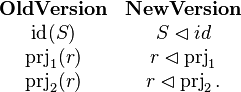$\begin{matrix} \textbf{Old Version} & \textbf{New Version}\\ \id(S) & S\domres id\\ \prjone(r) & r\domres\prjone\\ \prjtwo(r) & r\domres\prjtwo . \end{matrix}$


Moreover, in the case where the parameter is not needed, then it can be dropped altogether: no domain restriction is needed. For instance, to express that a relation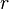$r$ is irreflexive, one would now write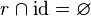$r\binter\id = \emptyset$.

## Partition

A new partition predicate is introduced. It is intended to provide an easier way to enter enumerated sets, while getting rid of the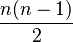$\frac{n(n-1)}{2}$ axioms needed to express pairwise distinctness (or disjointness). The partition operator is defined as follows: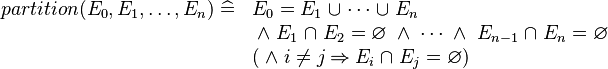$\begin{array}{ll} partition(E_0, E_1, \ldots, E_n)\defi & E_0 = E_1\bunion \cdots\bunion E_n \\ & \;\land\; E_1\binter E_2=\emptyset \;\land\;\cdots \;\land\; E_{n-1}\binter E_n = \emptyset \\ & (\;\land\; i \ne j \limp E_i \binter E_j = \emptyset ) \\ \end{array}$


where the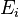$E_i$ are expressions bearing the same type.

Then, we can enter into a context :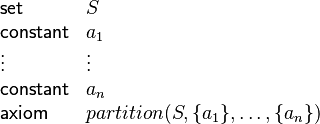$\begin{array}{ll} \mathsf{set} & S\\ \mathsf{constant} & a_1\\ \vdots & \vdots\\ \mathsf{constant} & a_n\\ \mathsf{axiom} & partition(S, \{a_1\}, \ldots, \{a_n\}) \end{array}$


which is a particular case of a set being defined by listing all its elements.

TODO

## Operator Associativity

Operators used to build sets of relations or functions, viz.

• relation
• total relation
• surjective relation
• total surjective relation
• partial function
• total function
• partial injection
• total injection
• partial surjection
• total surjection
• bijection

have no more relative priorities and loose associativity. Instead, users have to make it explicit by entering parenthesis in formulas.